# e 09, 201 (6) 2 points An equation for the level curve of f(z, y) = In(z+y) that passes through the point (0, e2) i...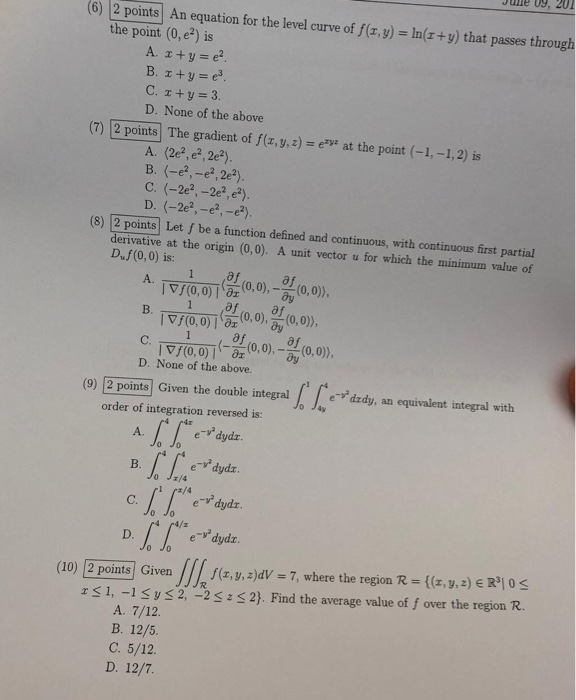e 09, 201 (6) 2 points An equation for the level curve of f(z, y) = In(z+y) that passes through the point (0, e2) is A. z + y = e2 B. I+y e C. z+y 3. D. None of the above (7) 2 points The gradient of f(z,y, z) = ep at the point (-1,-1,2) is A. (2e2,e2,2e2). B. (-e,-e,2e2). C. (-2e2,-2e2, e) D. (-2e2,-e,-e) (8) 2 points Let f be a function defined and continuous, with continuous first partial derivative at the origin (0,0). A unit vector u for which the minimum value of Duf (0,0) is: af A. Vf(0,0) z,0),(0,0)), af B. 1 C. D. None of the above. edzdy (9) 2 points Given the double integral equivalent integral with , an order of integration reversed is: 4x - dydr A. e dydz B. Jz/4 cz/4 e dydz. C. 0 Jo c4/z dydz y D. e" 0 f(x,y, z)dV = 7, where the region R = {(z,y, 2) E R| 0 S (10) 2 points Given R 2, -22

Q.7.) Gradient of the given function at given point.

☆ If we want to know the direction of any object, we draw the normal vector from that we come to know that what is the direction of the object,

☆ And if we want to know the direction of the function we use Gradient concept,

☆ Calulating gradient of any function at any point means we calculating its normal at that point from which we come to know the direction of the function at that particular point.

Find -:At point (-1,-1, 2)Q.9.)Changing the order of integration.

☆ Changing the order of integration means,

As we can see in the question, it is in the form,we have to integrate first w.r.t dx and then integrate it with dy,

☆ but in this method we reverse it order by, first we integrate it with respect to dy then w.r.t dx.

☆ this method makes most of the integration easy to solve.

For this we have to simply interchange the limit value, in the form☆ Now, let's start solving the question☆ It's limit are,☆ For changing the order, we first have to draw the limit on the graph and then change its order,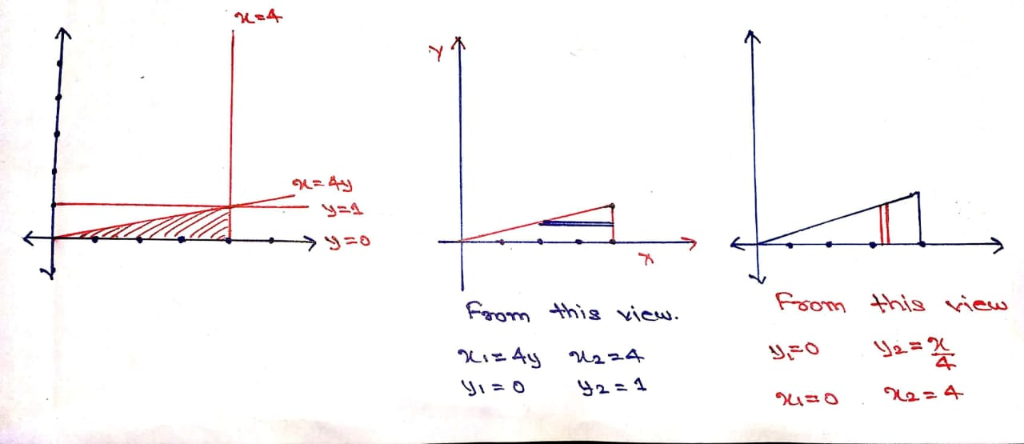I.) In the below figure, left most figure shows the correct diagram of the limit,

II.) In second figure, the limit is taken first with x as dependent variable and y as independent variable, which is mention in the question.

☆ For reversing the order of the limit, we just have to choose y as dependent variable and x as independent variable.

III.) In rightmost figure, the limit is taken as y as dependent figure and x as independent variable.

☆ The integral will look like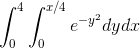☆ The answer which is in option is completely wrong, I had solve all 4 integral which is in the option and all are wrong the form and I had obtain the answer with the right concept.

☆☆ Please comment below if you have any doubt regarding this question before rating this answer.

#### Earn Coin

Coins can be redeemed for fabulous gifts.

Similar Homework Help Questions
• ### Question 6 6 pts Suppose that f(x, y, z) is a scalar-valued function and F(x, y,...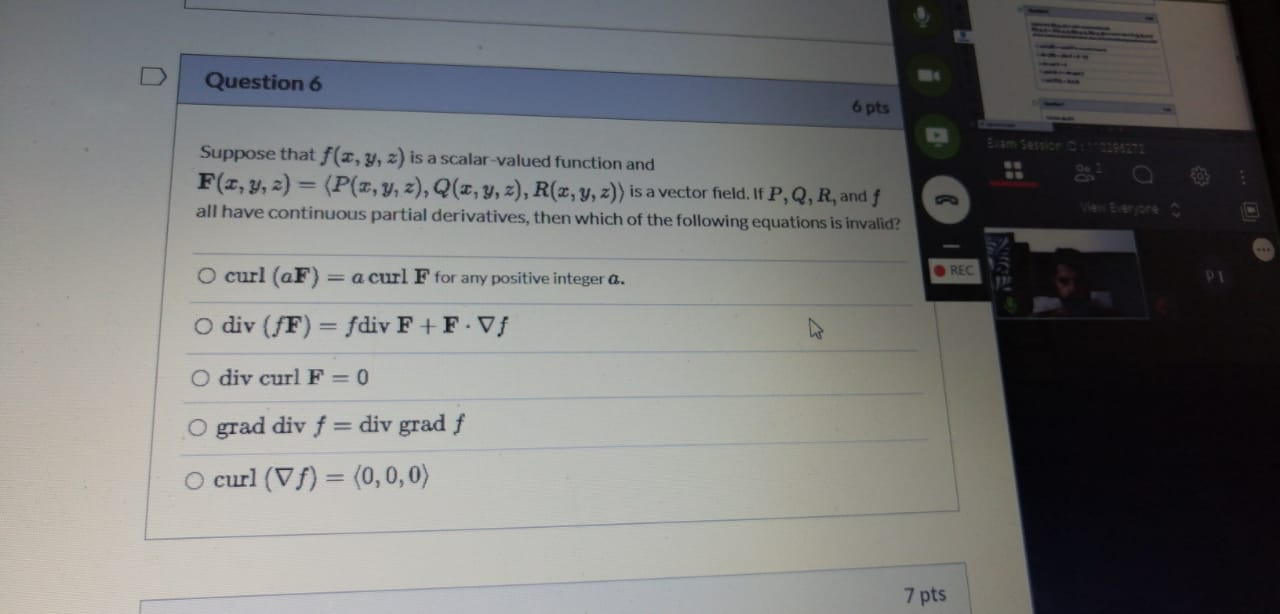Question 6 6 pts Suppose that f(x, y, z) is a scalar-valued function and F(x, y, z) = (P(x, y, z), Q(2,y,z), R(x, y, z)) is a vector field. If P, Q, R, and f all have continuous partial derivatives, then which of the following equations is invalid? O curl (aF) N21 = a curl F for any positive integer Q. REC o div (fF) = fdiv F+FVF Odiv curl F = 0 O grad div f = div grad...

• ### (8) 2 points Let f be a function defined and continuous, with continuous first partial derivative at the origin (0,...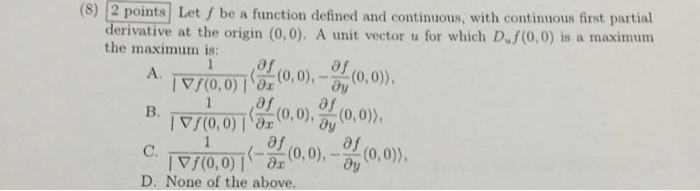(8) 2 points Let f be a function defined and continuous, with continuous first partial derivative at the origin (0,0). A unit vector u for which D.f (0,0) is the maximum is: maximum a 1 (0,0)), A. /(0,0)x,0),y (0 af B. (0,0) 8x0,0),(0,0)), af 1 ((0,0),-y C. (0,0), /(0,0) D. None of the above. (8) 2 points Let f be a function defined and continuous, with continuous first partial derivative at the origin (0,0). A unit vector u for which...

• ### Please answer fully and clearly. Thank you. )if (e-) (0.0)) if (z, y)#10, 0), 2. Consider f R2R defined by f(,) plHP, 0, if (x,y) (0,0)J (a) Show by explicit computation that the directional derivati...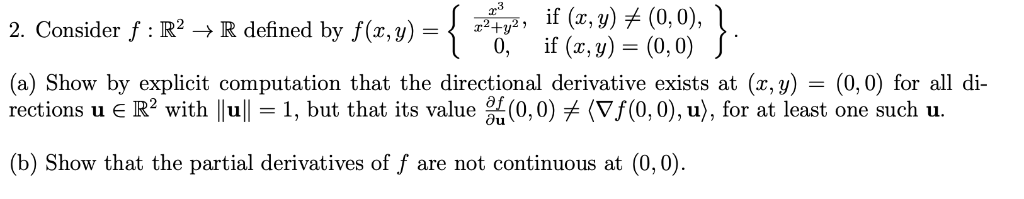Please answer fully and clearly. Thank you. )if (e-) (0.0)) if (z, y)#10, 0), 2. Consider f R2R defined by f(,) plHP, 0, if (x,y) (0,0)J (a) Show by explicit computation that the directional derivative exists at (x, y) - (0,0) for all di rections u R2 with lull-1, but that its value (0,0)メ(Vf(0,0), u), for at least one such u. b) Show that the partial derivatives of f are not continuous at (0,0) )if (e-) (0.0)) if (z, y)#10,...

• ### true or false is zero. F 9. The plane tangent to the surface za the point (0,0, 3) is given by the equation 2x - 12y -z+3-0. 10. If f is a differentiable function and zf(x -y), then z +. T 11. If...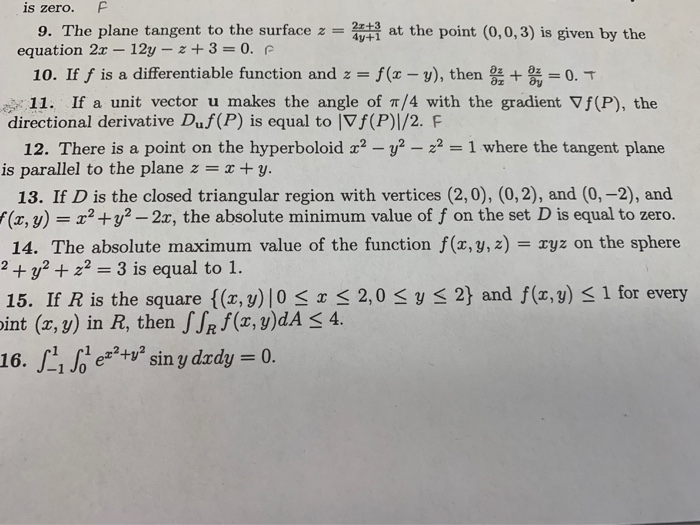true or false is zero. F 9. The plane tangent to the surface za the point (0,0, 3) is given by the equation 2x - 12y -z+3-0. 10. If f is a differentiable function and zf(x -y), then z +. T 11. If a unit vector u makes the angle of π/4 with the gradient ▽f(P), the directional derivative Duf(P) is equal to |Vf(P)I/2. F 12. There is a point on the hyperboloid 2 -y is parallel to the plane...

• ### b) i. Using e-8 definition show that f is continuous at (0,0), where f(x,y) = {aš...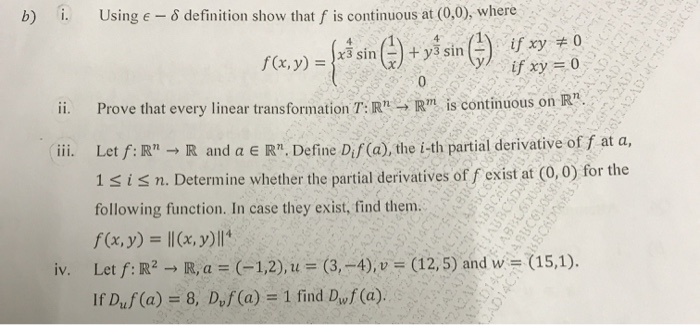b) i. Using e-8 definition show that f is continuous at (0,0), where f(x,y) = {aš sin () + yś sin () if xy + 0 242ADES if xy = 0 ii. Prove that every linear transformation T:R" - R" is continuous on R". iii. Let f:R" → R and a ER" Define Dis (a), the i-th partial derivative of f at a, 1 sisn. Determine whether the partial derivatives of f exist at (0,0) for the following function. In...

• ### Evaluate the line integral f F dr for the vector field F(x, y, z) curve C...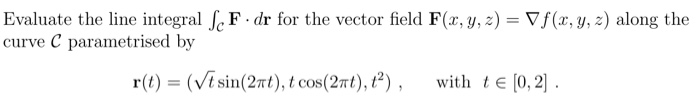Evaluate the line integral f F dr for the vector field F(x, y, z) curve C parametrised by Vf (x, y, z) along the with tE [0, 2 r() -(Vt sin(2πt), t cos (2πi), ?) , Evaluate the line integral f F dr for the vector field F(x, y, z) curve C parametrised by Vf (x, y, z) along the with tE [0, 2 r() -(Vt sin(2πt), t cos (2πi), ?) ,

• ### (2) Let f(z, y)-xy +x-y be defined on the closed disk {(z, y) E R2 :...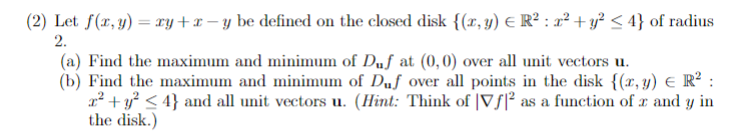(2) Let f(z, y)-xy +x-y be defined on the closed disk {(z, y) E R2 : z? + y2 < 4} of radius 2. (a) Find the maximu and minimu of Duf at (0,0) over all unit vectors u. (b) Find the maximum and minimum of Duf over all points in the disk(,y) E R2 r2 + y2 < 4} and all unit vectors u. (llint. Think of IvJF as a function ofェand y in the disk.)

• ### if (r.y) (0,0), 0,f (, y) (0, 0) 2. Consider f : IR2 -R defined by f(r,y)-+ (a) Show by explicit computation that the directional derivative exists at (x, y)- (0,0) for all oi rections u є R2 with 1...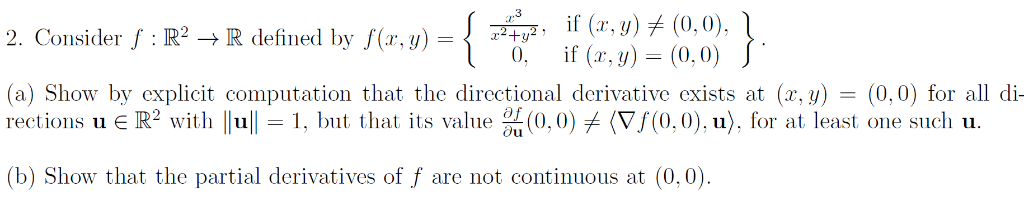if (r.y) (0,0), 0,f (, y) (0, 0) 2. Consider f : IR2 -R defined by f(r,y)-+ (a) Show by explicit computation that the directional derivative exists at (x, y)- (0,0) for all oi rections u є R2 with 1 11-1, but that its value %(0.0) (Vf(0,0).u), fr at least one sucli u. (b) Show that the partial derivatives of f are not continuous at (0,0) if (r.y) (0,0), 0,f (, y) (0, 0) 2. Consider f : IR2 -R...

• ### 1. (2 points) Find F dF if curl(F) 3 in the region defined by the 4 curves and C4 Ci F . d7 where F(x,y,z)-Wi +pz? + Vi> and C consists of the arc of the 2. (2 points) Evaluate curve y = sin(...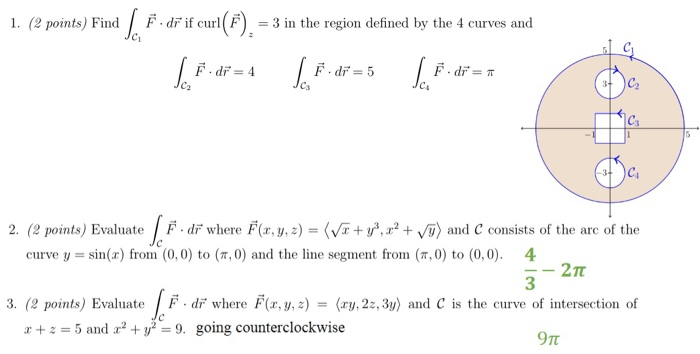1. (2 points) Find F dF if curl(F) 3 in the region defined by the 4 curves and C4 Ci F . d7 where F(x,y,z)-Wi +pz? + Vi> and C consists of the arc of the 2. (2 points) Evaluate curve y = sin(x) from (0,0) to (π, 0) and the line segment from (π,0) to (0,0). 4 3 3. (2 points) Evaluate F di where F.y,(ry, 2:,3) and C is the curve of intersection of 5 and y29. going...

• ### 1. (You may use the fact that 2xy ++ y2 for all real 2 and y.)...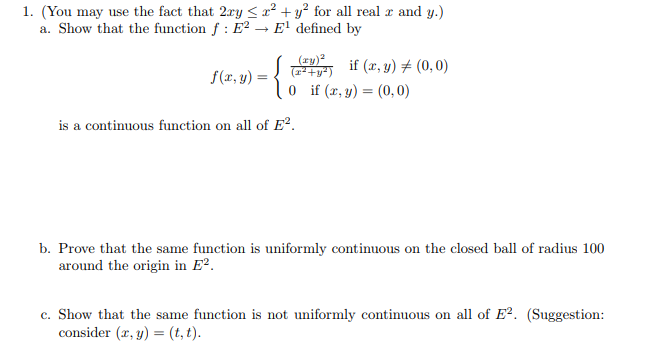1. (You may use the fact that 2xy ++ y2 for all real 2 and y.) a. Show that the function f : E→ El defined by f(x, y) = y) if (r, y) + (0,0) 10 if (2,y) = (0,0) is a continuous function on all of E. b. Prove that the same function is uniformly continuous on the closed ball of radius 100 around the origin in E2. c. Show that the same function is not uniformly continuous...